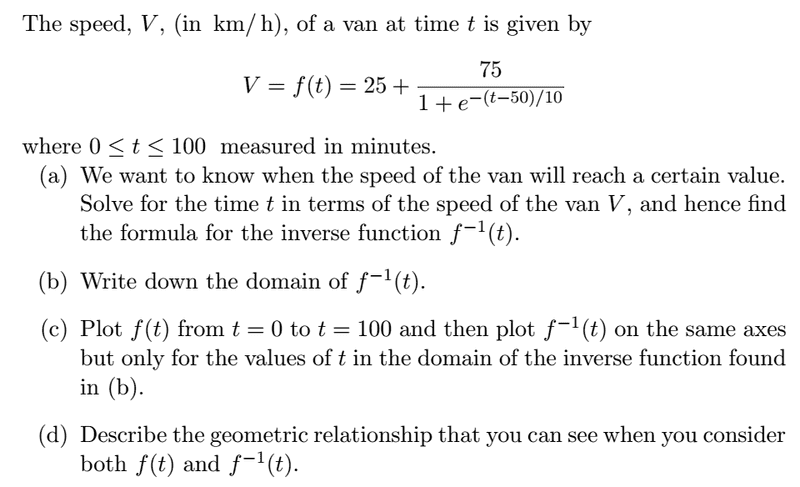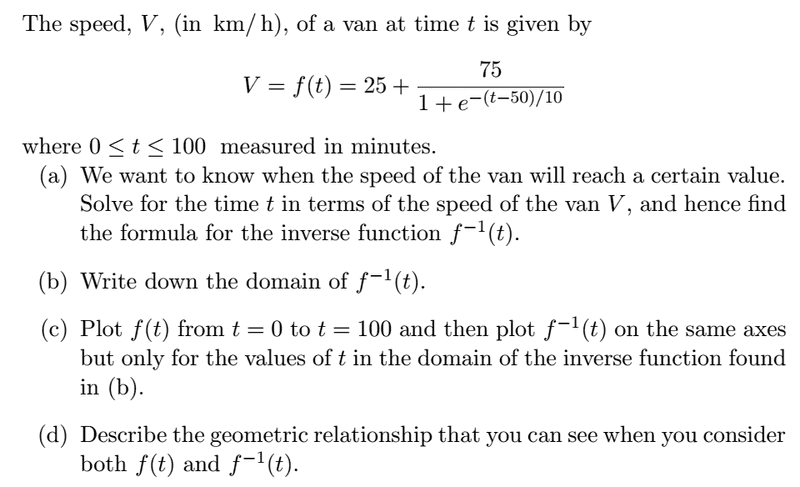# Shouldn't f(t) = V have inverse f^{-1} (V) not f^{-1} (t)?

• kostoglotov
In summary, the student is unsure if the inverse function is written as f^{-1} (V) or f^{-1} (t). The tutor has written to the student's instructor to ask if the inverse function is written as f^{-1} (V) or f^{-1} (t). The tutor is not confident in their answer and wants to get confirmation.f

## Homework Statement

I'm actually a tutor, and a student has shown me one of their assignments. I think there's a notation error, I'm quite confident there is actually, but I don't want to assert this, or have my student go asserting it, without some more certainty.

This is the questionNow, shouldn't the inverse function be written $f^{-1} (V)$?

I've written to the guy saying

"They've said solve for t in terms of V to find the inverse function...now, since there are only two variables, V and t, and inverting any relationship between them would just be finding one in terms of the other, f^{-1} is t as a function of V, so they should write it as f^{-1} (V)...right? I mean, you need to input V into the inverse function to find t...so therefore the inverse function is a function of V. So it should be written as f^{-1} (V). That's what I'd say anyway, I'm not a PhD in math, so I don't want to get over-confident about it.

It's not a big deal, and I'm sure the tutors and lecturers know perfectly well what they're doing, I'd say it would be a typo, but it could potentially lead to some confusion or misunderstanding in some instances...if I'm correct :)"

There has to be a domain change right? It makes no sense for the inverse to be in terms of t.

## The Attempt at a Solution

An inverse function is a function that undoes the action of the another function. A function g is the inverse of a function f if whenever y = f ( x ) then x = g ( y ) . In other words, applying f and then g is the same thing as doing nothing.

function is F(t) .v=F(t) ,the inverse of f is g then t=g(v) then if g is an inverse of f then application of g.f on a function say h should retain the original function
g.f ( h) = h
naturally g= f ^-1

an example

The inverse of a function has all the same points as the original function, except that the x's and y's have been reversed. . For instance, supposing your function is made up of these points: { (1, 0), (–3, 5), (0, 4) }. Then the inverse is given by this set of point: { (0, 1), (5, –3), (4, 0) }.

## Homework Statement

I'm actually a tutor, and a student has shown me one of their assignments. I think there's a notation error, I'm quite confident there is actually, but I don't want to assert this, or have my student go asserting it, without some more certainty.

This is the questionNow, shouldn't the inverse function be written $f^{-1} (V)$?

I've written to the guy saying

"They've said solve for t in terms of V to find the inverse function...now, since there are only two variables, V and t, and inverting any relationship between them would just be finding one in terms of the other, f^{-1} is t as a function of V, so they should write it as f^{-1} (V)...right? I mean, you need to input V into the inverse function to find t...so therefore the inverse function is a function of V. So it should be written as f^{-1} (V). That's what I'd say anyway, I'm not a PhD in math, so I don't want to get over-confident about it.

It's not a big deal, and I'm sure the tutors and lecturers know perfectly well what they're doing, I'd say it would be a typo, but it could potentially lead to some confusion or misunderstanding in some instances...if I'm correct :)"

There has to be a domain change right? It makes no sense for the inverse to be in terms of t.

## The Attempt at a Solution

I agree with you, but I have also seen it done both ways in different sources.

Hi,
As a physicist I agree with you: the range of V(t) has a different dimension so I wouldn't want to plot t(V) with V on the abscissa and t on the ordinate. Instead I would want to simply swap the x and y axes. And that's of course the idea behing the math of this exercise: that you get a nice kind of symmetry wrt y=x.

But, as a physicist, I find it rather fishy-- quite appropriate if I look at the picture resulting from the (c) part of the exercise !

I actually don't think there's anything wrong with the phrasing in the question in regards to finding the inverse function. You just switch $t$ for $V$ in the main function and solve for t...but you probably knew that already so this point I made is most likely moot.Given some invertible function, f :

Being asked to compare (on the same set of axes) the graph ##\ y = f(x)\ ## with the graph ##\ y = f^{-1}(x)\ ##, makes perfect sense.

In the example in this thread,it makes less sense due to the fact that there are different units involved with the two axes.

It's a mere coincidence that domain and range are over similar intervals numerically. Use seconds and meters per second and use the same "domain" for both graphs, and you won't see much on one of them.

Given some invertible function, f :

Being asked to compare (on the same set of axes) the graph ##\ y = f(x)\ ## with the graph ##\ y = f^{-1}(x)\ ##, makes perfect sense.

In the example in this thread,it makes less sense due to the fact that there are different units involved with the two axes.
Along the same lines with your objection about different units, there are the conversion formulas for Celsius <--> Fahrenheit:
C = (5/9)(F - 32), and
F = (9/5)C + 32

The first equation gives Celsius temp as a function of temp in °F, and the second gives the Fahr. temp as a function of the temp in °C, so obviously the units are different. If you switch the variables, you get a horrible mess.

A big gripe I have with a lot of the precalc textbooks is the emphasis they put on switching the variables when finding the inverse of a function. Many students misunderstand, and after switching the variables, think they have found the inverse, but have completely missed the most important step of actually finding the inverse relationship between the two variables.

Later, in calculus courses, the important skill is being able to take, say y = f(x), and write the equivalent equation, x = f-1(y), assuming that both f and f-1 are 1-to-1, and so, invertible. Being able write the inverse as y = f-1(x) is of just about zero importance in such applications as changing the order of integration in iterated integrals, or even changing from vertical strips to horizontal strips in ordinary integrals.

It's nice to recognize that the graphs of y = f(x) and y = f-1(x) are reflections of one another across the line y = x, but IMO, this pales in comparison to recognizing that y = f(x) and x = f-1(y) are equivalent equations; that is, both equations describe exactly the same set of points.
SammyS said:
It's a mere coincidence that domain and range are over similar intervals numerically. Use seconds and meters per second and use the same "domain" for both graphs, and you won't see much on one of them.

to compare (on the same set of axes) the graph ## y = f(x)## with the graph ## y = f^{-1}(x)## , makes perfect sense.
Yes and no. As others have remarked, the domains are different. The two x symbols there represent different variables, and you would in general need to use differently marked axes to plot them in the same paper space.
I think the confusion arises because on the one hand we think of the x and y there as the actual variables, and on the other as standing for the conventional co-ordinates in a Cartesian plot. It would be clearer to consider the original problem as plotting V=f(t) with V on the Y axis and t on the X axis, which makes y=f(x); then plotting it with V on the X axis and t on the Y axis, which makes the graph ## y = f^{-1}(x)##, but substituting back with the new association we find it represents ## t = f^{-1}(V)##.